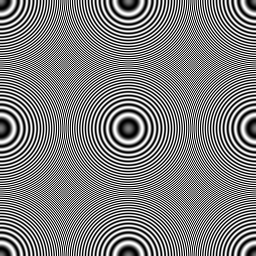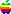# Open Source Repository

courtesy of
Ken Turkowski

The source code available from this page may be freely downloaded and used in any applications for any purpose, as long as the code is used in its entirety and the copyright notice and warranty information is retained.

If you make any improvements to this software, you should provide me with said improvements.

If any of this code is incorporated into a commercial product, you should notify me of this by email, and provide me with a complimentary copy of said product. :-)

## Index

Polynomial Roots
Inverse Square Root
Cube Root
Complex Roots of a Quadratic Equation
Real Roots of a Cubic Equation
Complex Roots of a Polynomial Equation
Fixed-Point Square Root
Nonlinear Functions
Real Roots of a Scalar Nonlinear Function
Real Roots of a System of Nonlinear Functions
Fixed-Point Trigonometric Functions using CORDIC
Linear Algebra
Linear Transformations
Null Space, EigenVectors
Miscellaneous Numerical Functions
Machine-independent I/O for IEEE Floating-Point Numbers
8-Bit Unsigned Floating-Point
Image Processing
Zone Plate Test Pattern
Image Rotation by ±90°, 180°
QuickTime VR
Matrix to Display a Movie within a Panorama

### Inverse Square Root

This is useful for normalizing vectors, because it eliminates divisions. It is faster that extracting the square root and reciprocating because only as much precision is computed as needed. This was inspired by a Weitek Technical note, was coded into C by me, and was published by me in Graphics Gems V, Academic Press.

 ``float InvSqrt(float x);``

InvSqrt.c

### Cube Root

This is an efficient methods for computing the cube root of a number in single precision. I wrote this code to assist in computing quadratic forward differencing for image warps.

 ``float CubeRoot(float x);``

CubeRoot.c

This finds the complex roots of a quadratic equation using an algorithm that is more robust than the usual one.

 ``long FindQuadraticRoots(const float coeff, float re, float im);``

### Real Roots of a Cubic Equation

This will find the real roots of a cubic equation with real coefficients.

 ``long FindCubicRoots(const float coeff, float x);``
FindCubicRoots.c

### Complex Roots of a Polynomial Equation with Real Coefficients

This will find all of the roots of a polynomial equation with real coefficients.

I converted this to C from the Fortran code in ACM algorithm #30, Numerical Solution of the Polynomial Equation, written by K. W. Ellenberger.

 ````void FindPolynomialRoots(` ` const float *a, /* Coefficients */` ` float *u, /* Real component of each root */` ` float *v, /* Imaginary component of each root */` ` float *conv, /* Convergence constant associated with each root */` ` long n, /* Degree of polynomial (order-1) */` ` long maxiter, /* Maximum number of iterations */` ` long fig /* The number of decimal figures to be computed */` `);````

FindPolynomialRoots.c

### Roots of a Nonlinear Function

This finds the roots of an arbitrary function. An initial guess is given for each one.

I converted this to C from ACM Algorithm # 25, Real Zeros of an Arbitrary Function, written by B. Leavenworth.

 ````long FindZerosOfFunction(` ` float (*func)(float), /* The function whose zeros are to be found */` ` float *root, /* The roots */` ` long n, /* The number of roots sought */` ` long maxiter, /* Maximum number of iterations */` ` float epsr, /* Relative convergence criterion on successive iterates */` ` float epsf, /* Absolute convergence criterion on the function values */` ` float epsmr, /* Closeness of multiple roots (see mrspr) */` ` float mrspr /* The spread for multiple roots, that is,` ` * if |rt - root[i]| < epsmr,` ` * where root[i] is a previously found root,` ` * then rt is replaced by rt + mrspr. */` `);````

FindZerosOfFunction.c

### Fixed Point Square Root

This finds the square root of a fixed point number with 30 fractional bits.

 ``Fract FractSqrt(Fract a);``

FractSqrt.c

### Nonlinear System of Equations

This will solve a nonlinear system of equations.

I converted this to C from Algol from ACM Algorithm #316, Solution of Simultaneous Non-Linear Equations, written by K. M. Brown.

 ````long SolveNonlinearSystem(` ` float (**func)(float*), /* Vector of functions */` ` float *x, /* Vector of independent variables */` ` long n, /* Order of system */` ` float numsig, /* Number of significant decimal digits */` ` float *maxit /* Maximum number of iterations; return actual number */` `);````

SolveNonlinearSystem.c

### Fixed-Point Trigonometric Functions using CORDIC

This module computes a variety of trigonometric functions in fixed-point, using CORDIC iterations.

I wrote this after reading the seminal paper by Walther.

 ````void FxUnitVec(long *cos, long *sin, long theta);` `void FxRotate(long *argx, long *argy, long theta);` `long FxAtan2(long x, long y);` `void FxPolarize(long *argx, long *argy);````

`FxUnitVec()` generates the sine and cosine of the given angle theta.

`FxRotate()` rotates the given vector by the angle theta. A vector can be converted from polar to rectangular coordinates by setting `argx` to the radius and `argy` to zero.

`FxAtan2()` returns the angle of the vector (x,y).

`FxPolarize()` converts rectangular coordinates to polar coordinates. The radius is returned in `argx`, and the angle in `argy`.

Cordic.c

### General Purpose Linear Transformations

This general-purpose workhorse for linear transformations with arbitrary sized matrices is nearly as efficient as special-purpose routines written for fixed sized matrices. I wrote this because I wanted to take advantage of the efficiency afforded by pointers rather than array indices, in part to simulate dedicated hardware to perform matrix multiplication.

`void LinearTransform(real *L, real *R, real *P, long nRow, long lCol, long rCol);`

### `Examples`

`LinearTransform(v, v, d, 1, 3, 1);` Squared norm of a 3-vector, yielding a scalar.
`LinearTransform(u, v, d, 1, 3, 1);` Dot product of two 3-vectors, yielding a scalar.
`LinearTransform(u, v, d, 3, 1, 3);` Tensor product of two 3-vectors, yielding a 3x3 2-rank tensor.
`LinearTransform(v, M, w, 1, 3, 3);` Transforms a 1x3 row vector by a 3x3 matrix, yielding a 1x3 row vector.
`LinearTransform(V, M, W, 16, 4, 4);` Transforms 16 1x4 row vectors by a 4x4 matrix, yielding 16 1x4 row vectors.
`LinearTransform(V, T, U, 3, 4, 3);` Multiplies a 3x4 matrix by a 4x3 matrix yielding a 3x3 matrix.

LinearTransform.c

### Null Space of a Square Matrix

This procedure, to find the null space of a square matrix, is especially useful for finding the eigenvectores corresponding to a particular eigenvalue.

I converted this to C from ACM Algorithm 270, Finding Eigenvectors by Gaussian Elimination, written by Albert Newhouse, University of Houston.

 `long NullSpace(const float *a, float *result, float eps, long n);`

NullSpace.c

### Machine-independent I/O for IEEE floating point numbers

These routines provide machine-independent I/O for IEEE floating point numbers in single-, double- and extended-precision formats (32, 64, and 80 bits, respectively), irrespective of the actual floating-point format used on a given machine.

These routines were written by me and Malcolm Slaney, primarily to support the AIFF sampled sound format, which makes use of IEEE extended precision floating point format, as well as single and double precision.

This has been used on a wide variety of platforms, including the Cray, DEC, and IBM mainframes, which did not use IEEE floating-point as its native floating-point format, as well as Intel's x86, Motorola's 680x0, Motorola PowerPC, and MIPS' microprocessors, which do use IEEE floating-point. Note that the extended IEEE format is not found on many IEEE-compliant processors, yet these routines can recover their values to the full precision supplied by a given machine's highest precision floating point.

 ````defdouble ConvertFromIeeeSingle( char *bytes);` `defdouble ConvertFromIeeeDouble( char *bytes);` `defdouble ConvertFromIeeeExtended(char *bytes);` ` ` `void ConvertToIeeeSingle( defdouble num, char *bytes);` `void ConvertToIeeeDouble( defdouble num, char *bytes);` `void ConvertToIeeeExtended(defdouble num, char *bytes);````

ToFromIEEE.c

### 8-Bit Unsigned Floating-Point

Sometimes there is a need for a small data type with a large range, where the range is more important than the precision. This module implements several floating-point formats that fit into an 8-bit byte. The interesting formats have a significand of 4, 5, or 6 bits.

• 4 significand bits yields a range of 507904 ~ 524287 = 2^19-1
• 5 significand bits yields a range of 4032 ~ 4095 = 2^12-1
• 6 significand bits yields a range of 508 ~ 511 = 2^ 9-1

 ```/* These provide basic conversion to/from integers */ unsigned long ToIntFromUFloat8(UFloat8 u, unsigned long sigBits); UFloat8 FromIntToUFloat8(unsigned long i, unsigned long sigBits);``` ```/* These provide basic conversion to/from single precision floating-point */ float ToFloatFromUFloat8(UFloat8 u, unsigned long sigBits); UFloat8 FromFloatToUFloat8(float f, unsigned long sigBits);``` ```/* These provide L1 normalized vectors */ void NormalizedL1FloatVectorFromUFloat8(unsigned long n, unsigned long sigBits, const UFloat8 *u, float *f); void NormalizedL1UCharVectorFromUFloat8(unsigned long n, unsigned long sigBits, const UFloat8 *u, unsigned char *c); ```

UFloat8.c

### Zone Plate

The circular zone plate has zero horizontal and vertical frequencies at the center. Horizontal frequencies increase as you move horizontally, and vertical frequencies increase as you move vertically.

These patterns are useful to:

• evaluate image compression
• evaluate filter properties
• evaluate the quality of resampling algorithms
• adjust gamma correction - at the proper gamma setting, the møires should be minimized

This code was written by me and Steve Gabriel from a paper published by the BBC. We also implemented the hyperbolic zone plate, which is easier to implement, but not as intuitive to use.ZonePlate -w 256 -h 256 -o z128 -s 128 This one covers all representable frequencies.ZonePlate -w 256 -h 256 -o z256 -s 256 This one goes beyond the previous one until the first alias. Note the gray vertical bands resulting from analog video signal filtering in the display system. No such filtering occurs vertically, so there are no corresponding horizontal bands. An annoying flicker appears instead on an interlaced display.

ZonePlate.c

### Image Rotation by ±90°, 180°

These procedures will rotate an image by ±90° or 180°, in a general image setting, where the pixel size if 8, 16, 24, or 32 bits, and the source and destination are different. A convenience wrapper is provided to specialize this for GWorlds, as found in QuickTime on the Macintosh, Windows, etc.

 ````void RotateImage90CW(` ` void *src, long srcRowBytes,` ` void *dst, long dstRowBytes,` ` long width, long height,` ` long bytesPerPixel` ` );` `void RotateImage90CCW(` ` void *src, long srcRowBytes,` ` void *dst, long dstRowBytes,` ` long width, long height,` ` long bytesPerPixel` ` );` `void RotateImage180(` ` void *src, long srcRowBytes,` ` void *dst, long dstRowBytes,` ` long width, long height,` ` long bytesPerPixel` ` );` `void RotateGWorld(GWorldPtr srcGW, GWorldPtr dstGW, long degrees);````

RotateGWorld.c

### Compute 3x3 Matrix to Immerse an Image or Movie into a QuickTime VR Panorama

With QuickTime 4.0, a high-precision renderer is used to display QuickTime VR panoramas. This module will generate a 3x3 floating-point matrix that can be used to perform a projective transformation on an image or movie, so that the image appears fixed with respect to the panorama as the user pans, tilts, and zooms around the panorama.

 ````void ImmerseImageInQTVR(` ` GWorldPtr imageGW, float imagePan, float imageTilt, float imageFOV,` ` GWorldPtr viewGW, float viewPan, float viewTilt, float viewFOV,` ` float *M` `);````

ImmerseImageInQTVR.c

## Other Sources of Open Sources

• Panorama Tools, Helmut Dersch's free panorama authoring plugins (Mac, Windows, Linux)

[QuickTime VR Home Page |QuickTime Home Page |Apple Home Page]

last revised: 7/25/05